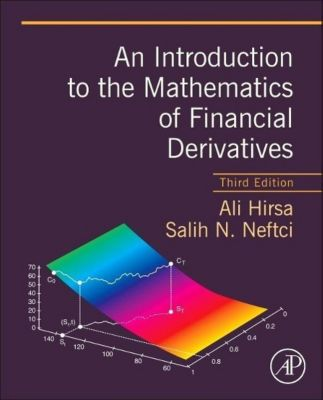# An Introduction to the Mathematics of Financial Derivatives

(Sprache: Englisch)Merken
Teilen
Merken
Teilen
An Introduction to the Mathematics of Financial Derivatives is a popular, intuitive text that eases the transition between basic summaries of financial engineering to more advanced treatments using stochastic calculus. Requiring only a basic knowledge of...
lieferbar
versandkostenfrei

Bestellnummer: 55925033

Buch93.50
• Kauf auf Rechnung
• Kostenlose Rücksendung
• Ratenzahlung möglich
lieferbar
versandkostenfrei

Bestellnummer: 55925033

Buch93.50
An Introduction to the Mathematics of Financial Derivatives is a popular, intuitive text that eases the transition between basic summaries of financial engineering to more advanced treatments using stochastic calculus. Requiring only a basic knowledge of...

## 0 Gebrauchte Artikel zu „An Introduction to the Mathematics of Financial Derivatives“

ZustandPreisPortoZahlungVerkäuferRating
• Kauf auf Rechnung
• Kostenlose Rücksendung
• Ratenzahlung möglich